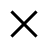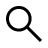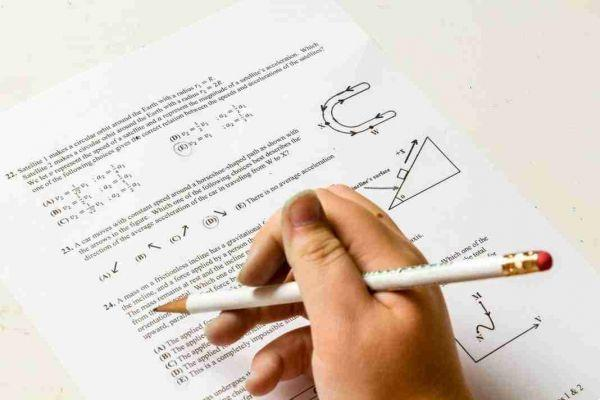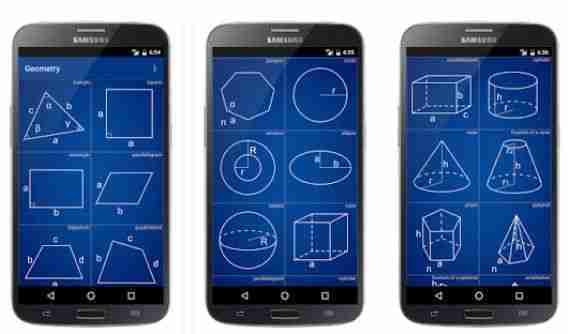Buscar

# Apps for solving math problems: the best for Android and iOSWho I am
Lluis Enric Mayans
@lluisenricmayansHow complicated can it be to solve a math problem made by expressions, geometric calculations and many other variables.

With these free apps for solving math problems, perhaps it will be easier for you to find the solution.

Now we are going to list the best ones for you App for solving math problems available for Android and iOS.

## App for solving math problems

### Photomath – Calcolatrice

Just point the camera at the math expression and PhotoMath will magically display the result with step-by-step detailed instructions.

Photomath offre:
∙ Camera calculator
∙ Handwriting recognition (NEW)
∙ Step-by-step instructions
∙ Smart calculator
• Charts (NEW)

Photomath supports: arithmetic, integers, fractions, decimal numbers, roots, algebraic expressions, linear equations / inequalities, quadratic equations / inequalities, absolute equations / inequalities, systems of equations, logarithms, trigonometry, exponential and logarithmic functions, derivatives and integrals.

### MyScript Calculator

https://youtu.be/V5IBkH-gNlo

Easy, simple and intuitive, just write the math expression on the screen then let the MyScript technology perform its magic symbols and transform numbers into digital text and deliver the result in real time.

The same experience as writing on paper with the advantages of a digital device (Scratch-out, results in real time,…).

- You can write any arithmetic formula by hand.
- Write and solve mathematical expressions in an intuitive and natural way, without using the keyboard.
- Erase gestures to easily delete symbols and numbers.
- Horizontal and vertical orientation.
- Undo and redo functions.

### Solve Expressions

Solve Expressions allows you to solve numerical and literal expressions, with fractions and powers, showing all the steps!

Write your expression in the appropriate field, you can check from the preview if the text is correct, press Solve and the steps will be shown below.

### Geometry CalculatorCalculate areas and solid figures:
Triangle, square, rectangle, parallelogram, rhombus, trapezoid, rectangle, polygon, circle, circle, ellipse.
Sphere, cube, box, cylinder, cone, truncated cone, prism, pyramid, truncated pyramid, octahedron.